# Common Core: 1st Grade Math : Composing Three-Dimensional Shapes

## Example Questions

### Example Question #1523 : Common Core Math: Grade 1

What two shapes can you find in this shape?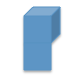A cube and a trapezoid

A cube and a triangle

A cube and a square

A cube and a circle

A cube and a square

Explanation: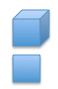### Example Question #1524 : Common Core Math: Grade 1

What two shapes can you find in this shape?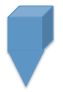A cube and a rectangle

A cube and a trapezoid

A cube and a square

A cube and a triangle

A cube and a triangle

Explanation: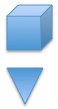### Example Question #1525 : Common Core Math: Grade 1

What two shapes can you find in this shape?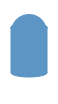A rectangle and circle

A cylinder and a triangle

A cylinder and a circle

A rectangle and a triangle

A cylinder and a circle

Explanation: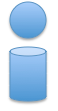### Example Question #1526 : Common Core Math: Grade 1

The sides of a rectangular prism are made up of __________.

Trapezoids

Circles

Rectangles

Triangles

Rectangles

Explanation:

The sides of a rectangular prism are made up of rectangles.### Example Question #1527 : Common Core Math: Grade 1

The base, or bottom, of a cylinder is made of up what shape?

Circle

Square

Rectangle

Triangle

Circle

Explanation:

The base, or bottom, of a cylinder is made up of a circle.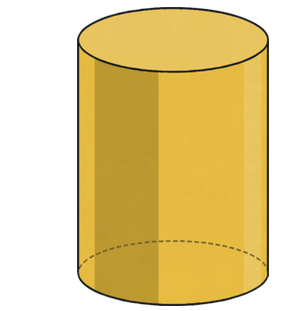### Example Question #1528 : Common Core Math: Grade 1

The sides of a cube are made up of ____________.

Rectangles

Triangles

Squares

Rectangles and squares

Squares

Explanation:

A cube is a 3-dimensional shape with 6 sides. Each side of a cube is a square. Thus, the sides of a cube are made up of squares.### Example Question #1529 : Common Core Math: Grade 1

Using the clues below identify the three-dimensional shape.

• I have 6 flat surfaces.
• Each flat surface is a square.
• I have no curved surfaces.

What am I?

A sphere

A cube

A cone

A cylinder

A cube

Explanation:

The three-dimensional figure being described in the riddle is a cube. A cube, like a cardboard box or a die from a board game, has six sides, each side is flat, each side is a square, and there are no curved sides. The other answer choices include curved sides and do not have six flat surfaces.

### Example Question #1530 : Common Core Math: Grade 1

Andy takes two cubes and stacks them on top of each other. What three-dimensional shape did he create?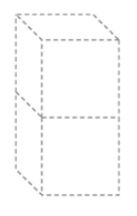Pyramid

Rectangular Prism

Cube

Cylinder

Rectangular Prism

Explanation:

Andy created a rectangular prism by stacking two cubes on top of each other. A rectangular prism has six flat surfaces, six sides, and four identical faces that are larger than a pair of smaller faces. Each flat surface on a rectangular prism is a square or rectangular shape.

### Example Question #51 : Geometry

Using the clues below identify the three-dimensional shape.

• I have five flat surfaces.
• Four of my surfaces are triangles.
• I have no curved surfaces.

What am I?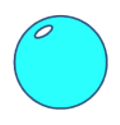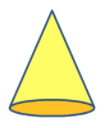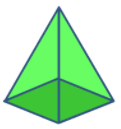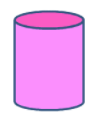Explanation:

The three-dimensional figure that is being described in the riddle is a pyramid. A pyramid has four surfaces that are triangles, no curves surfaces, and a total of five flat surfaces. The base of the pyramid in the example is a square. The other figures have curved surfaces and do not match the clues.

### Example Question #52 : Geometry

Using the clues below identify the three-dimensional shape.

• I have zero flat surfaces.
• I have zero faces.
• I have no edges.

What am I?